Aknowledgments: François Husson class on youtube, the book “R pour la statistique et la science des données”.

# Load all packages needed to execute the job
# If the packages are not installed, write
# install.packages("<name of package>")

library(ggplot2) # plot

# Clear any existing variables
rm(list = ls())

# Set seed for reproducibility
set.seed(123)

# Principal component analysis

## Illustrative example

Before going into details, let us look at a funny example. Imagine that I generate two variables $$X_1$$ and $$X_2$$ from normal distributions. We want these variables to be linked (correlated) and such that $$X_j \sim \mathcal{N}(0,1)$$. The following chunk performs the simulation. You can take the output data frame and explore the data first with univariate analysis. And then with a bivariate plot.

Remark: An outlier is in the dataset. Can you recover it?

library(MASS) # for simulations
## Warning: package 'MASS' was built under R version 4.1.2
Sigma <- matrix(c(1,0.8,1,0.8),2,2)
simulated_data <- mvrnorm(n = 500, mu = c(0,0), Sigma)
output <- data.frame(simulated_data)
names(output) <- c("X1", "X2")
output[501,] <- c("X1" = 2, "X2" = -2) # outlier step

Solution

library(ggplot2)
ggplot(output, aes(y = X1)) +
geom_boxplot() +
theme_classic()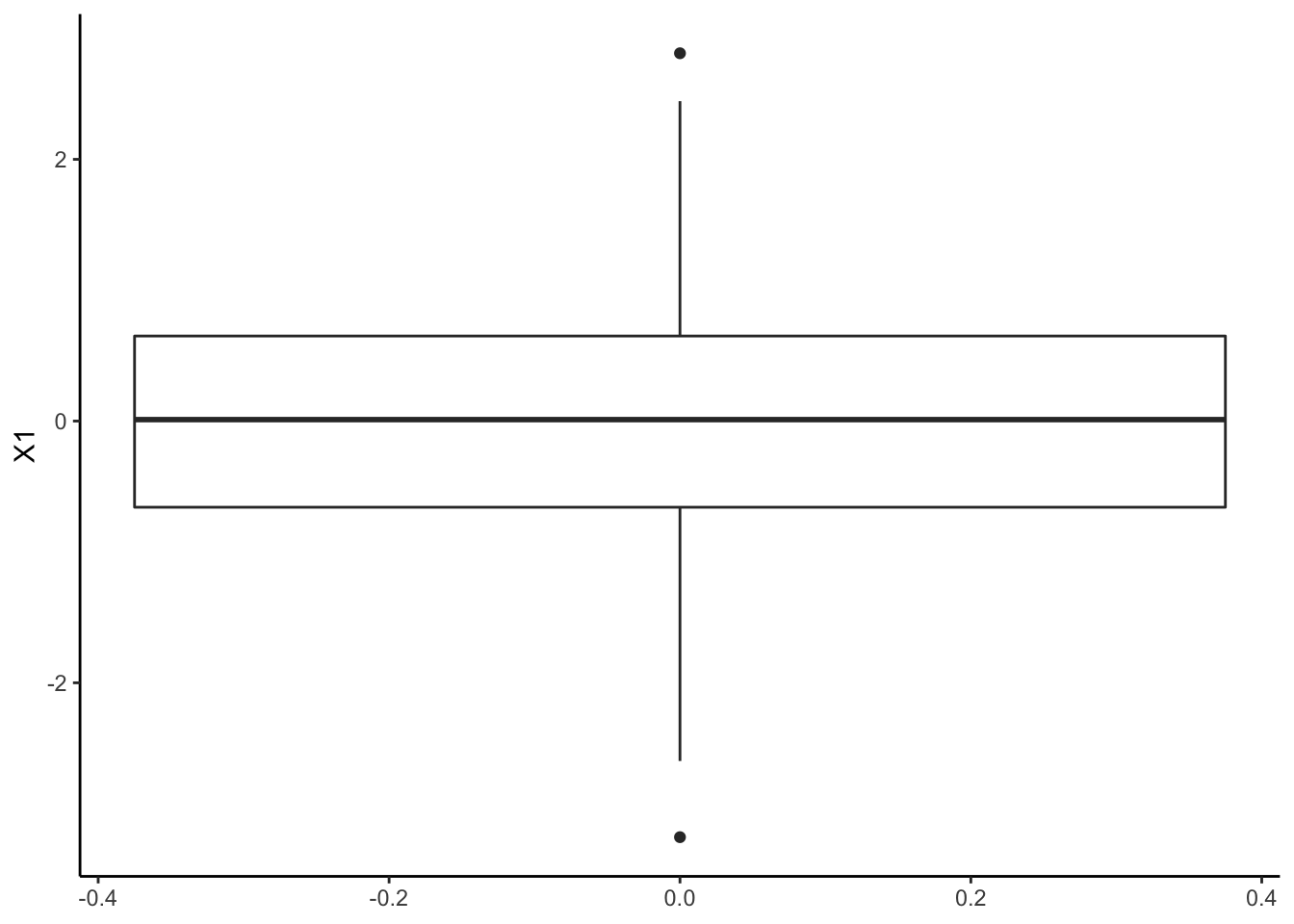ggplot(output, aes(y = X2)) +
geom_boxplot() +
theme_classic()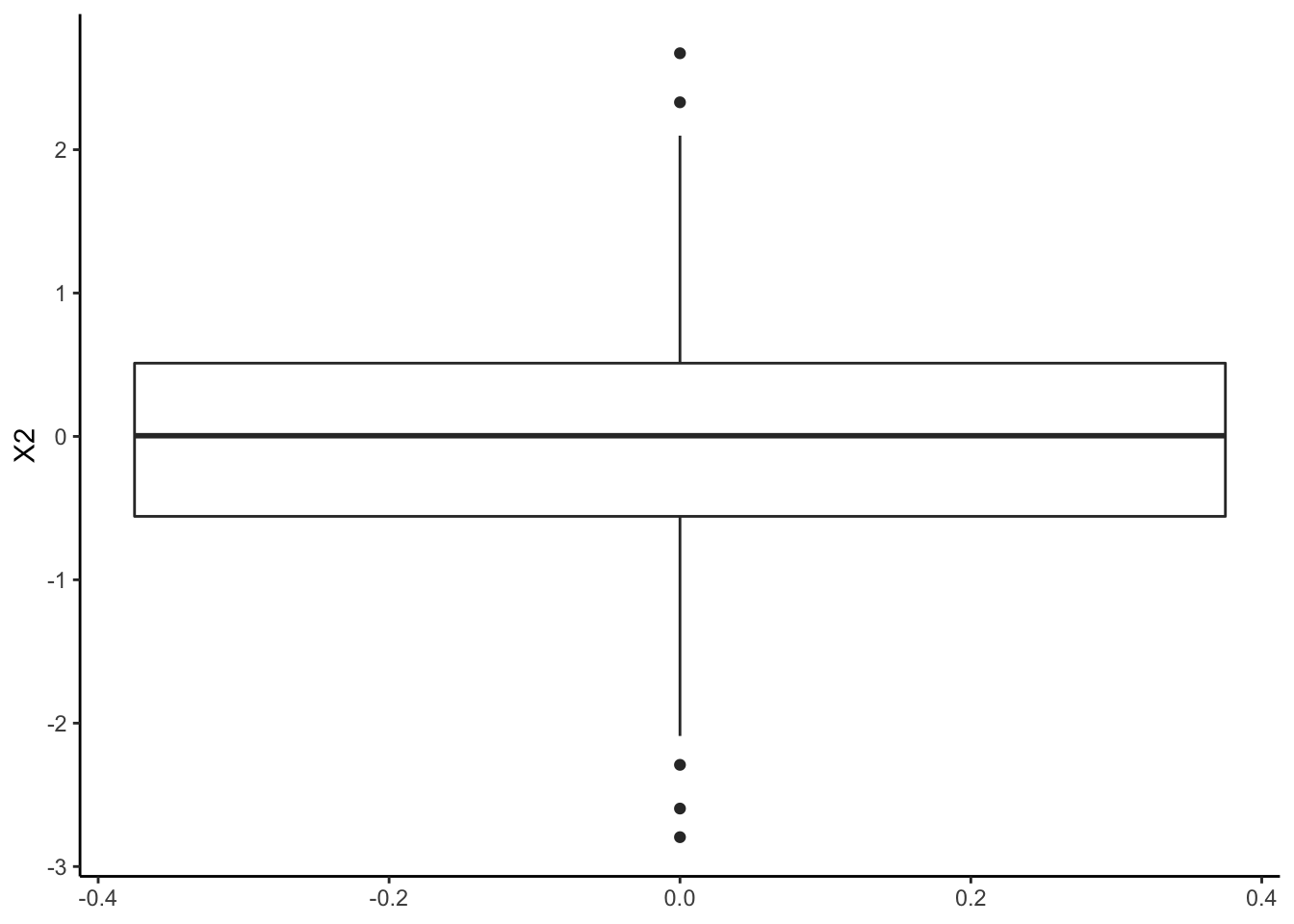ggplot(output, aes(x = X1)) +
geom_histogram(bins = 20, fill = "blue", alpha = 0.6, color = "grey") +
theme_classic()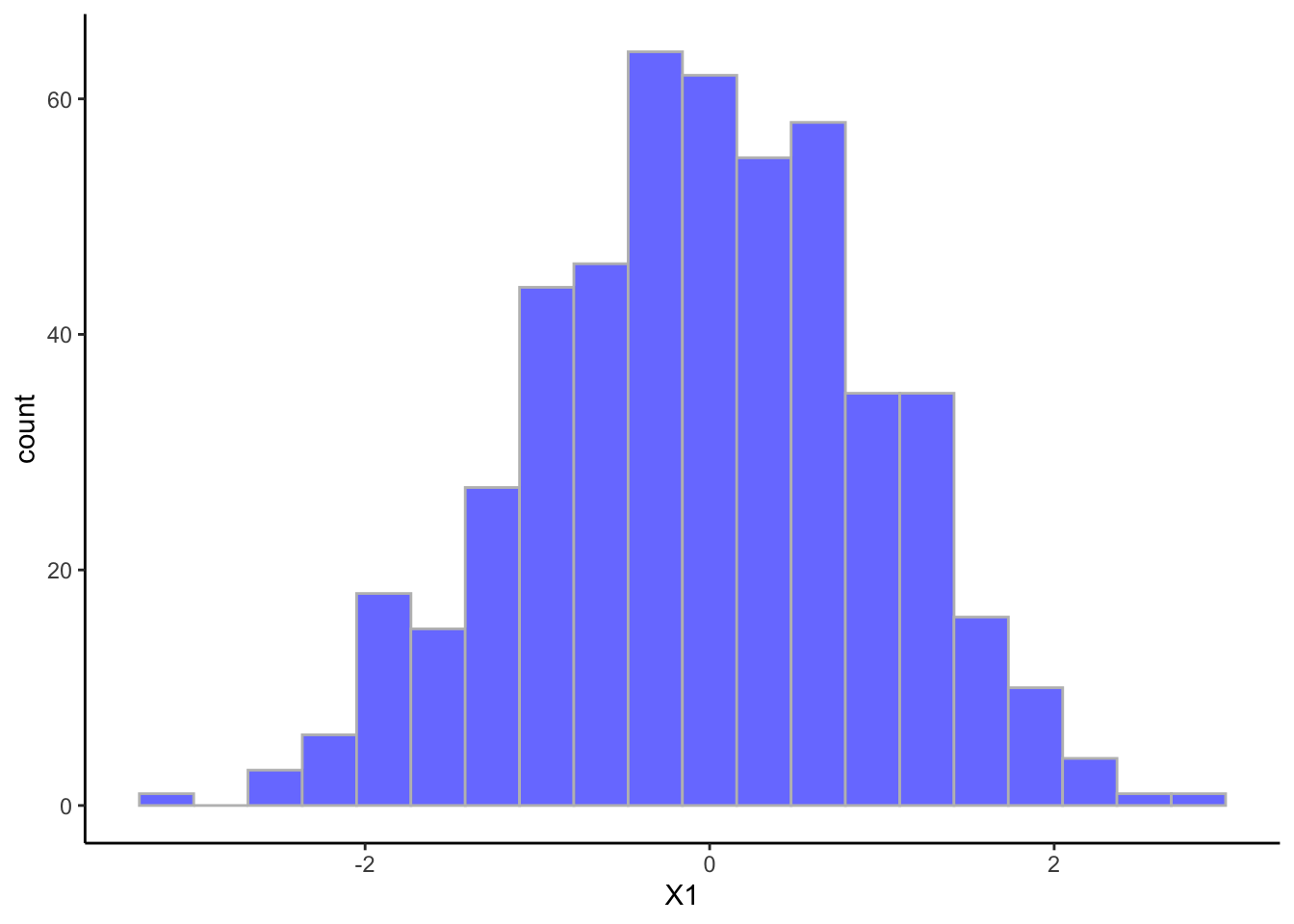ggplot(output, aes(x = X2)) +
geom_histogram(bins = 20, fill = "blue", alpha = 0.6, color = "grey") +
theme_classic()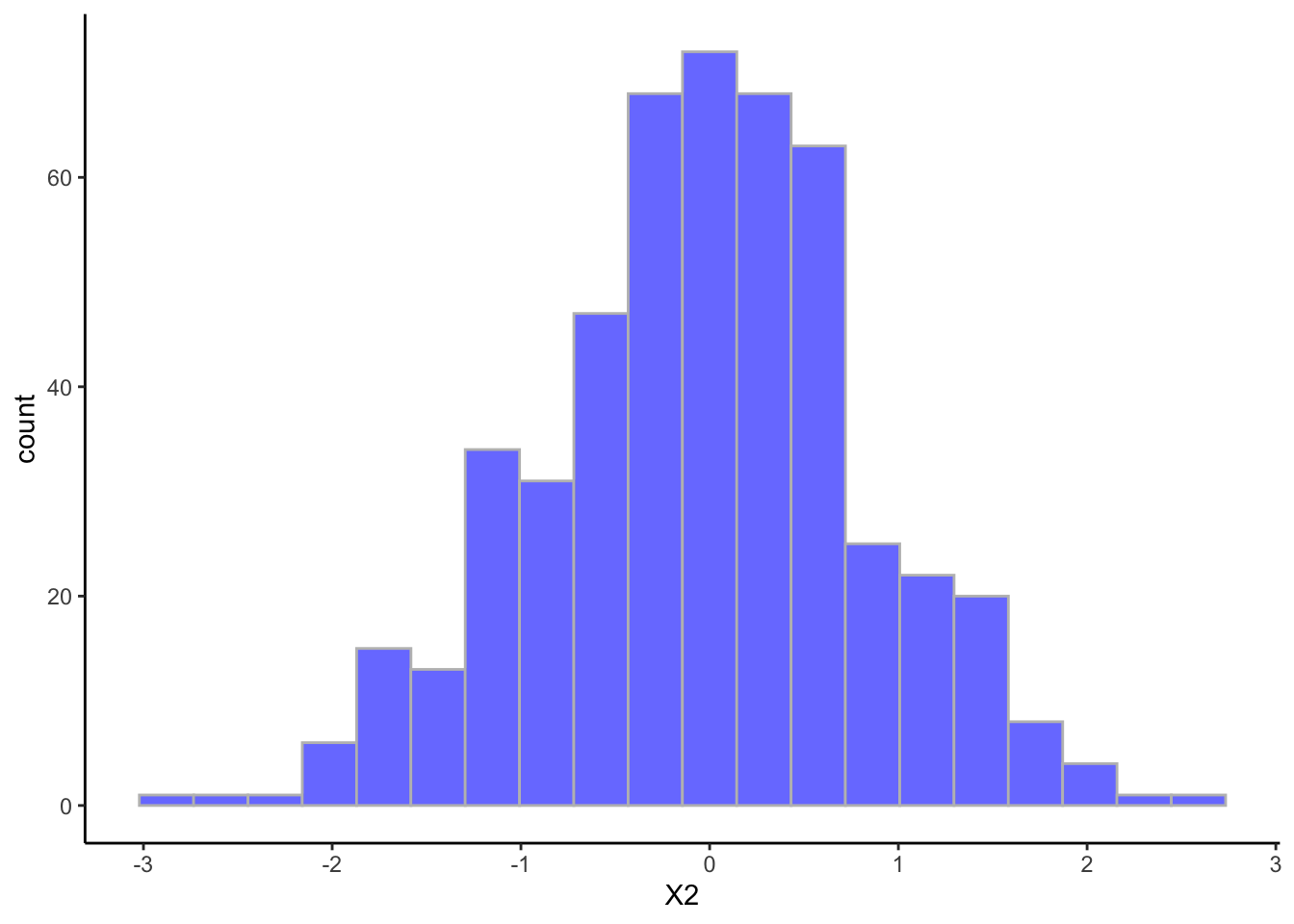ggplot(output, aes(x = X1, y = X2)) +
geom_point() +
theme_classic()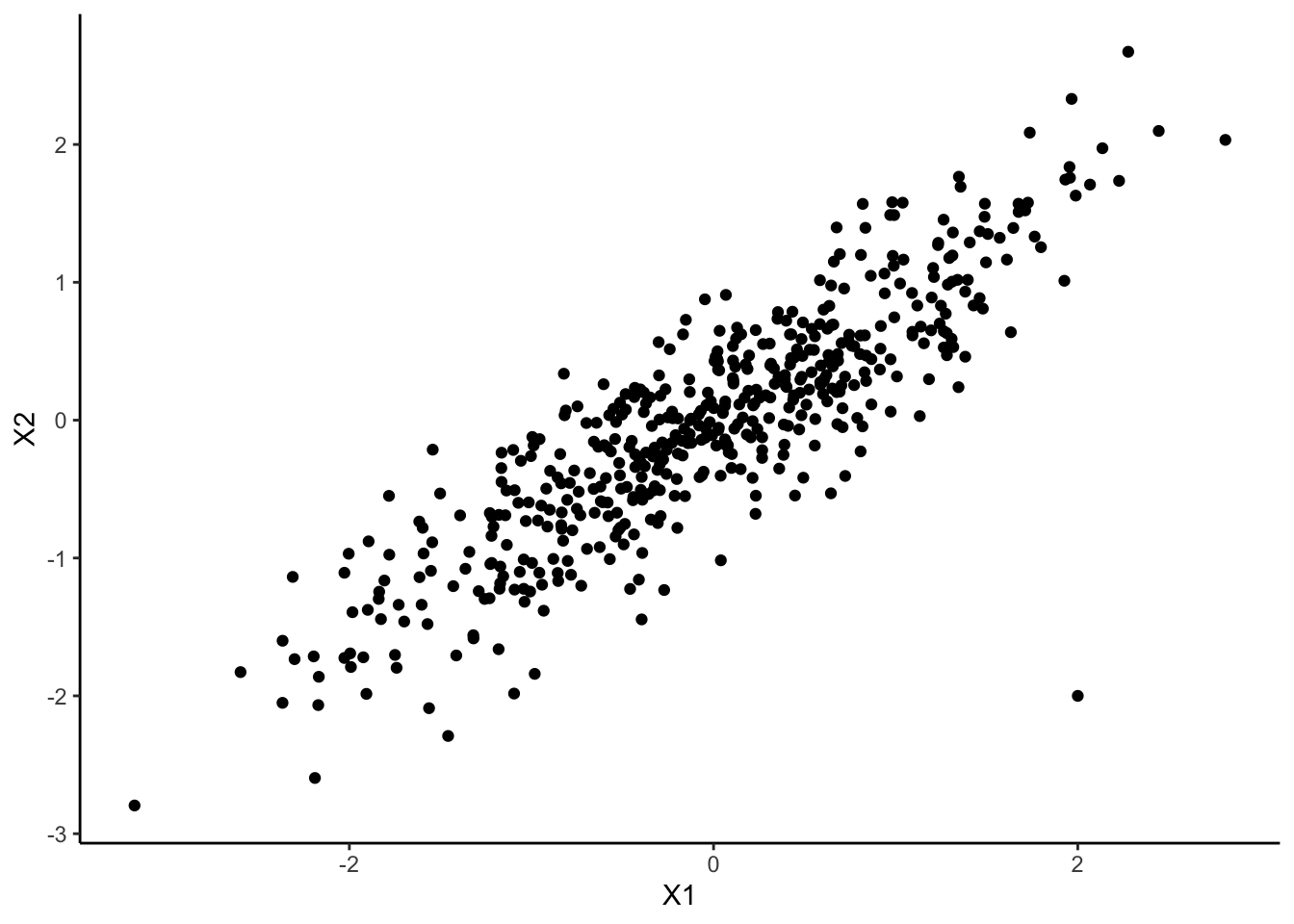The outlier is clearly identifiable on this scatter plot, but not using only the boxplot or any univariate tool. This is to highlight that PCA will allow us to see high dimensional outliers. In other words, PCA will allow you to observe multidimensional outliers.

End of solution

## General introduction

Context

Principal Component Analysis (usually the shortname is PCA but you can also find ACP in French) focuses on typical data you can find in several domains: observations (or individus) in rows, and variables in column. Note that the PCA focuses on quantitative variables (for example age, or price, but not color or sex). For example we can study the average temperature depending on cities. In that case cities are rows, and in column the average temperature per month.

A typical question you may ask on your data is: how much the different observations are close to one another considering the variables? (remember that everything you will conclude depends on these variables that you added in your initial model) You can also see PCA as a way to find a low-dimensional representation that captures the “essence” of high-dimensional data

What can you interpret from data?

The PCA will group similar individuals together. Information are also learned on variables, with the correlated variables (meaning that you have a linear link between two variables), and also which variables synthetize the most the observations, or which variables bring different informations.

Package

In this notebook we propose to use the package and the function .

## An example: the decathlon data set

The data set is based on the decathlon results during the Athene’s olympic games and the Décastar (another competition). For each athletes the data set contains the results in the 10 tests, with the total number of points and ranking. The competition in which the athlete participated is also mentioned.

For both competitions, the following information is available for each athlete: performance for each of the 10 events, total number of points (for each event, an athlete earns points based on performance; here the sum of points scored), and final ranking. The events take place in the following order: 100 meters, long jump, shot put, high jump, 400 meters (first day) and 110 meter hurdles, discus, pole vault, javelin, 1500 meters (second day).

The overall objective of this exercice is to characterize the athletes and their differences, and to observe it tests evaluate similar skills or different ones. The aim of conducting PCA on this dataset is to determine profiles for similar performances: are there any athletes who are better at endurance events or those requiring short bursts of energy, etc? And are some of the events similar? If an athlete performs well in one event, will he necessarily perform well in another?

### Question 1

First, load the data and inspect the data (for example which variables are quantitative or qualitative?).

Remark: This step is the first step you should do before any data analysis, and not only PCA.

Solution question 1

# Load data
decathlon <- read.csv(file = "decathlon.csv", row.names = 1)
dim(decathlon)
##  41 13
summary(decathlon)
##      X100m         Long.jump       Shot.put       High.jump         X400m
##  Min.   :10.44   Min.   :6.61   Min.   :12.68   Min.   :1.850   Min.   :46.81
##  1st Qu.:10.85   1st Qu.:7.03   1st Qu.:13.88   1st Qu.:1.920   1st Qu.:48.93
##  Median :10.98   Median :7.30   Median :14.57   Median :1.950   Median :49.40
##  Mean   :11.00   Mean   :7.26   Mean   :14.48   Mean   :1.977   Mean   :49.62
##  3rd Qu.:11.14   3rd Qu.:7.48   3rd Qu.:14.97   3rd Qu.:2.040   3rd Qu.:50.30
##  Max.   :11.64   Max.   :7.96   Max.   :16.36   Max.   :2.150   Max.   :53.20
##   X110m.hurdle       Discus        Pole.vault       Javeline
##  Min.   :13.97   Min.   :37.92   Min.   :4.200   Min.   :50.31
##  1st Qu.:14.21   1st Qu.:41.90   1st Qu.:4.500   1st Qu.:55.27
##  Median :14.48   Median :44.41   Median :4.800   Median :58.36
##  Mean   :14.61   Mean   :44.33   Mean   :4.762   Mean   :58.32
##  3rd Qu.:14.98   3rd Qu.:46.07   3rd Qu.:4.920   3rd Qu.:60.89
##  Max.   :15.67   Max.   :51.65   Max.   :5.400   Max.   :70.52
##      X1500m           Rank           Points     Competition
##  Min.   :262.1   Min.   : 1.00   Min.   :7313   Length:41
##  1st Qu.:271.0   1st Qu.: 6.00   1st Qu.:7802   Class :character
##  Median :278.1   Median :11.00   Median :8021   Mode  :character
##  Mean   :279.0   Mean   :12.12   Mean   :8005
##  3rd Qu.:285.1   3rd Qu.:18.00   3rd Qu.:8122
##  Max.   :317.0   Max.   :28.00   Max.   :8893

All the variables are quantitative except the competition type. Not that if you don’t add the item , then you will have an additional variable being the name of the participant. It is not a problem, but don’t forget to remove it when doing the PCA or else. Probably the simplest solution is to have it as row names. The commant also inform us on the number or observations. here we have 41 different observations.

End of solution question 1

### Question 2

Apply a PCA on the data using the function from FactoMineR, and interpret it.

Tips: - First install the package.

• The appropriate function is called PCA.

• You can check if this function does or not the normalization step going in the documentation ().

• Why are normalization and reduction an important step?

• Explain your choices for the active and illustrative variables/individuals? (because you don’t have to use all the variables to perform the PCA, you can only run it on a subset of variables that makes more sens.)

• When you interpret the data, you can also do a bar plot of the eigenvectors found by the PCA. For this purpose you can use the result object of the PCA analysis, and look at the component of this object. You can plot this using the function or ggplot2 (which is a little bit more challenging, but a good exercice)

Solution question 2

First, check that the package is installed and don’t forget to call the library. Usually in a notebook all the librairy calls are at the beginning of the notebook for more clarity. Also, it can be useful to recall why you call a library, so that you do not end up with a long list of packages where you don’t remember the purpose.

# Install the package
#install.packages("FactoMineR", dependencies = TRUE)

library(FactoMineR) # package for PCA
?PCA

Discussion on normalization

The normalization is an important step as it allows to compare all the variables with the same importance. For example imagine we have a data set with two variables A and B. The variable A is in kg and the other B in g, then the variable in g will count more in the distance. We recall that the distance between two observations is given with $$d = (a_i-a_j)^2 - (b_i-b_j)^2$$

Therefore if an identical difference in weights will be counted differently 0.2kg squarred or 200 squarred. Because in this data set the data have different unis we have no choice but to center the data and normalize it. Note that this is automatically done with the command.

In our specific example when the data is standardized, it is possible to compare two variables with different units and to say sentences such as “Paul is more remarkable by his performance on 100m than John is by is X400m”. With a value above 2, it means that the performance is way beyond average for example.

Which variables matter?

Only the result at each test matters. In fact, to obtain a typology of the athletes based on their performances for the 10 decathlon events, such as “two athletes are close since they have similar performance profiles”, the distances between two athletes are defined on the basis of their performances in the 10 events. Thus, only the performance variables are considered active; the other variables (number of points, rank, and competition) are supplementary. Here, the athletes are all considered as active individuals.

library(FactoMineR)
res.PCA <- PCA(decathlon, quanti.sup = c(11, 12), quali.sup = 13)
## Warning: ggrepel: 1 unlabeled data points (too many overlaps). Consider
## increasing max.overlaps